# KSEEB Solutions for Class 8 Maths Chapter 2 Algebraic Expressions Additional Questions

Students can Download Maths Chapter 2 Algebraic Expressions Additional Questions and Answers, Notes Pdf, KSEEB Solutions for Class 8 Maths helps you to revise the complete Karnataka State Board Syllabus and score more marks in your examinations.

## Karnataka State Syllabus Class 8 Maths Chapter 2 Algebraic Expressions Additional Questions

Question 1.
(a) Terms having the same literal factor with the same exponents are called
(A) exponents
(B) like terms
(C) factors
(D) unlike term
(B) like terms

(b) The coefficient of ab in 2ab is
(A) ab
(B) 2
(C) 2a
(D) 2b
(B) 2

(c) The exponential form of a x a x a is
(A) 3a
(B) 3 + a
(C) a3
(D) 3 – a
(C) a3

(d) Sum of two negative integers is
(A) negative
(B) positive
(C) zero
(D) infinite
(A) negative

(e) What should be added to a2 + 2ab to make it a complete square?
(A) b2
(B) 2ab
(C) ab
(D) 2a
(A) b2(f) What is the product of (x + 2) (x – 3)?
(A) 2x – 6
(B) 3x – 2
(C) x2 – x – 6
(D) x2 – 6x
(C) x2 – x – 6

(g) The value of (7.2)2 is (use an iden-tity to expand)
(A) 49.4
(B) 14.4
(C) 51.84
(D) 49.04
(C) 51.84

(h) The expansion of (2x – 3y)2is
(A) 2x2 + 3y2+ 6xy
(B) 4x2 + 9y2 – 12xy
(C) 2x2 +3y2 – 6xy
(D) 4x2 +9y2 + 12xy
(B) 4x2 + 9y2 – 12xy

(i) The product of 58 × 62 is (use an identity)
(A) 4596
(B) 2596
(C) 3596
(D) 6596
(C) 3596

Question 2.
Take away 8x – 7y – 8p + 10q from 10x + 10y – 7p + 9qQuestion 3.
Expand
(i) (4x + 3)2
(a + b)2 = a2 + 2ab + b2
Here a = 4x, b = 3
(4x + 3)2 = (4x)2 + 2.4×3 .+ 32
= 16x2 + 24x + 9

(ii) (x + 2y)2
(a + b)2 = a2 + 2ab + b2
Here a = x, b = 2y
(x + 2y)2 = x2 + 2.x.2y + (2y)2
= x2 + 4xy + 4y2

(iii) $$\left(x+\frac{1}{x}\right)^{2}$$
(a + b)2 = a2 + 2ab + b2
Here a = x, b = 1/xQuestion 4.
Expand
(i) (2t + 5) (2t – 5).
(a + b) (a – b) = a2 – b2
Here = a = 2t, b = 5 ]
(2t + 5) (2t – 5) = (2t)2 – 52
= 4t2 – 25

(ii) (xy + 8) (xy – 8)
(a + b) (a -b) = a2 – b2
Here a = xy, b = 8
(xy + 8) (xy – 8) = (xy)2 – 82
= x2y2 – 64

(ii) (2x + 3y) (2x – 3y)
(a + b) (a -b) = a2 – b2
Here = a = 2x, b = 3y
(2x + 3y) (2x – 3y) = (2X)2 – (3y)2 = 4x2 – 9y2Question 5.
Expand
(i) (n – 1) (n + 1) (n2 + 1)
(n – 1) (n + 1) (n2 + 1)
= (n2 – l2) (n2 + 1)
= (n2 – 1) (n2 + 1)
= (n2)2 – l2
= n4 – 1

(ii)$$\left(n-\frac{1}{n}\right)\left(n+\frac{1}{n}\right)\left(n^{2}+\frac{1}{n^{2}}\right)$$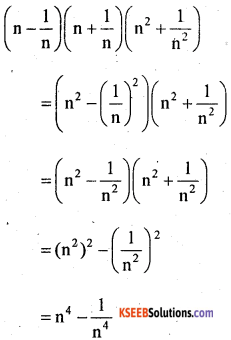(iii) (x – 1) (x + 1) (x2 + 1) (x4 + 1)
(x – 1) (x + 1) (x2 + 1) (x4 + 1)
= (x2 – l2) (x2 + 1) (x4 + 1)
= (x2 – 1) (x2 + 1) (x4 + 1)
= [(x2)2 – l2)] (x4 + 1)
= (x4 – 1) (x4 + 1)
= (x4)2 – l2
= X8 – 1

(iv) (2x – y) (2x + y) (4x2 + y2)
(2x – y) (2x + y) (4x2 + y2)
= [(2x)2 – y2) (4x2 + y2)
= (4x2 – y2) (4x2 + y2)
= [(4x2)2 – (y2)2)]
=16x4 – y4Question 6.
Use appropriate formulae and compute
(i) (103)2
1032 = (100 + 3)2
(a + b)2 = a2 + 2ab + b2
Here a = 100, b = 3
(100 + 3)2 = 1002 + 2.100.3 + 32
= 10000 + 600 + 9
1032 = 10609

(ii) (96)3
962 = (100 – 4)2
(a – b)2 = a2 – 2ab + b2
Here a = 100, b = 4
(100 + 3)2 = 1002 + 2.100.4 + 42
= 10000 – 800 + 16
1032 = 9216

(iii) 107 x 93
107 x 93 = (100 + 7)(100 – 7)
(a + b) (a – b) = a2 – b2
Here a = 100, b = 7
(100 + 7) (100 – 7) = 1002 – 72
= 10000 – 49 = 9951

(iv) 1008 x 992
1008 x 992 = (1000 + 8)(100 – 8)
(a + b) (a – b) = a2 – b2
Here a = 1000, b = 8
(1000 + 8) (100 – 8) = (1000)2 – 82
= 1000000 – 64
1008 x 992 = 999936

(v) 1852 – 1152
1852 – 1152
(a – b)2 = (a + b) (a – b)
Here a = 185, b = 115
1852 – 1152 = (185 + 115) (185 – 115)
= (300) (70)
1852 – 1152 = 21000

Question 7.
If x + y = 7 and xy = 12 find x2 + y2
(x + y)2 = x2 + 2xy + y2
(x + y)2 = x2 + y2 + 2xy
72 = x2 + y2 + 2(12)
[Substituting for x +y and xy]
49 = x2 + y2 +24
x2 + y2 = 49 24
x2 + y2 = 25

Question 8.
If x + y = 12 and xy = 32 find x2 + y2
(x + y)2 = x2 + y2 + 2xy
122 = x2 + y2 + 2(32)
[Substituting for x +y and xy]
144 = x2 + y2 + 64
x2 + y2 = 144 – 64
x2 + y2 = 80

Question 9.
If 4x2 + y2 = 40 and xy = 6 find 2x + y
4x2 + y2 = 40
(2x)2 + y2 = 40
(2x + y)2 = (2x)2 + y2 + 2.2x.y
(2x + y)2 = 4x2 + y2 + 4xy
= 40 + 4 x 6
= 40 + 24
(2x + y)2 = 64
2x + y = ±√64
2x + y = ±√8Question 10.
If x – y = 3 and xy = 10 find x2 + y2
(x – y)2 = x2 + y2 – 2xy
32 = x2 + y2 + 2(10)
[Substituting for x – y and xy]
9 = x2 + y2 – 20
9 + 20 = x2 + y2
∴ 29 = x2 + y2
x2 + y2 = 29

Question 11.
If $$x+\frac{1}{x}$$ = 3 find $$x^{2}+\frac{1}{x^{2}}$$ and $$x^{3}+\frac{1}{x^{3}}$$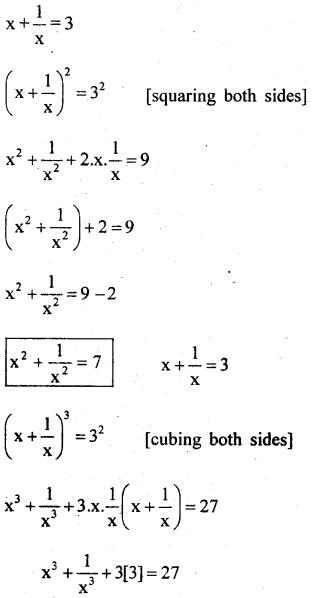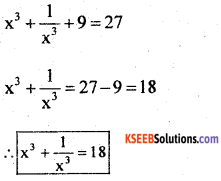Question 12.
$$x+\left(\frac{1}{x}\right)=6$$ find $$x^{2}+\frac{1}{x^{2}}$$ and $$x^{4}+\frac{1}{x^{4}}$$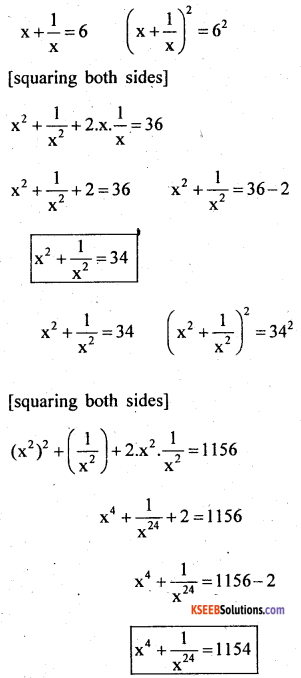Question 13.
Simplify (i) (x + y)2 + (x – y)2
(x + y)2 + (x – y)2
= x2 + y2 + 2xy + x2 + y2 – 2xy
= x2 + y2 + x2 + y2 = 2x2 + 2y2 ]
= 2(x2 + y2)

(ii) (x + y)2 x (x – y)2
(x + y)2 x (x – y)2
= (x2 + y2 + 2xy) (x2 + y2 – 2xy)
= x2 (x2 + y2 – 2xy) + y2 (x2 + y2 – 2xy) + 2xy (x2 + y2 – 2xy)
= x4 + x2y2 – 2x3y + x2y2 + y4 – 2xy3 + 2x3y + 2xy3 – 4x2y2
= X4 + 2x2y2 – 4x2y2 + y4
= x4 – 2x2y2 + y4Question 14.
Express the following as difference of two squares
(i) (x + 2z) (2x + z)
x(2x + z) + 2z (2x + z)
= 2x2 + xz + 4xz + 2z2
= 2x2 + 2z2 + 4xz + xz
= 2 (x2 + z2 + 2xz) + xz
= 2(x + z)2 + xz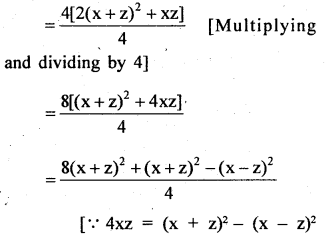(iii) 4(x + 2y) (2x + y)
4(x + 2y) (2x + y)
= 4(2x2 + xy + 4xy + 2y2)
= 4(2x2 + 2y2 + 4xy + xy)
=4 (2 (x2 + y2 + 2xy) + xy)
= 4 (2 (x + y)2 + xy)
= 8 (x + y)2 + 4xy
= 8 (x + y)2 + (x + y)2 – (x – y)2
[∵ 4xy = (x + y)2 – (x – y)2] = 9 (x + y)x^{4}+\frac{1}{x^{4}} – (x – y)2 = [3 (x + y)]2 – (x – y)2

(iii) (x + 98)( (x+ 102)
(x + 98)( (x + 102)
= (x + 100 – 2) (x + 100 + 2)
= (x + 100)2 – 22
[∵(a + b) (a – b) = a2 – b2]

(iv) 505 x 495
505 x 495 = (500 + 5) (500 – 5)
= (500)2 – 52

Question 15.
If a = 3x – 5y, b = 6x + 3y and c = 2y – 4x find
(i) a + b – c
a + b – c = 3x – 5y + 6x + 3y – (2y – 4x)
= 9x – 2y – 2y + 4x
= 13x – 4y

(ii) 2a – 3b + 4c = 2(3x – 5y) – 3 (6x + 3y) + 4 (2y – 4x)
= 6x – 10y – 18x – 9y + 8y – 16 x
= 6x – 18x – 16 x 10y – 9y + 8y
= 6x – 34x – 19y + 8y = – 28x – 11y
= – (28x + 11y)Question 16.
The perimeter of a triangle is 15×2 – 23x + 9 and two of its sides are 5×2 + 8x – 1 and 6×2 – 9x + 4. Find the third side
Let the sides of the triangle be a, b and c
Let a = 5x2 + 8x – 1,
b = 6x2 – 9x + 4
Perimeter = 15x2 – 23x + 9
a + b + c = 15x2 – -23x + 9 5x2 + 8x – 1 + 6x2 – 9x + 4 + c
= 15x2 – 23x + 9
llx2 – x + 3 + c <= 15x2 – 23x + 9
c = 15x2 – 23x + 9 – (llx2 – x + 3)
= 15x2 – 23x + 9 – llx2 + x – 3
c = 4x2 22x + 6
∴ The third side is 4x2 – 22x + 6

Question 17.
Two adjacent sides of a rectangle are 2x2 – 5xy + 3z2 and 4xy – x2 – z2. Find its perimeter.
Let l = 2x2 – 5xy + 3z2 and b = 4xy – x2 – z2
Perimeter of a rectangel = 2(l + b)
= 2 [2x2 – 5xy + 3z2 + 4xy – x2 – z2]
= 4x2 – lOxy + 6z2 + 8xy – 2x2– 2z2]
= 2x2 + 4z2 – 2xy

Question 18.
The base and altitutde of a triangle are (3x – 4y) and (6x + 5y) respectively. Find its area.
Area of a triangle = $$\frac{1}{2}$$ x base x height
= $$\frac{1}{2}$$ x(3x-4y)(5x + 5y)
= $$\frac{1}{2}$$[(3x(6x + 5y) – 4y(6x + 5y) ]
= $$\frac{1}{2}$$[18x2 +15y)-24y-20y2]
= $$\frac{1}{2}$$[18x2 – 9xy – 20y2]

Question 19.
The sides of a rectangle are 2x + 3y and 3x + 2y. From this a square of side length x + y is removed. What is the area of the remaining region.
Area of the remaining region
= Area of rectangle – Area of sqûare
= length x breadth – (side)2
= (2x + 3y) (3x + 2y) – (x + y)2
=2x(3x + 2y) + 3y(3x + 2y) – [x2 + y2 + 2xy]
= 6x2 + 4xy + 9xy + 6y2 – [x2 + y2 + 2xy]
= 6x2 + 13xy + 6y2 – x2 – y2 – 2xy = 5x2 + 11xy + 5y2

Question 20.
If a, b, c are rotational numbers such that a2 + b2 + c2 – ab – be – ca = 0 prove that a = b = c
a2 +b2 + c2 – ab – bc – ca = 0 Multiplying both the sides by 2
2(a2 + b2 + c2 – ab – be – ca) = 2 x 0
2a2 + 2b2 + 2c2 – 2ab – 2bc – 2ca = 0
a2 + a2 + b2 + b2 + c2 + c2 – 2ab – 2bc – 2ca = 0
a2 – 2ab + b2 + b2 – 2bc + c2 + c2 – 2ca + a2 = 0
(a – b)2 + (b – c)2 + (c – a)2 =0
(a – b)2 = 0, (b – c)2 = 0, (c – a)2 = 0 [v If the sum of positive quantities is equal to zero then each quantity is equal to zero]
∴ (a-b)2 = 0 ⇒ a – b = 0 ⇒ a = b
(b-c)2 = 0 ⇒ b – c = 0 ⇒ b = c
(c-a)2 = 0 ⇒ c – a = 0 ⇒ c = a
∴ a = b = c.error: Content is protected !!